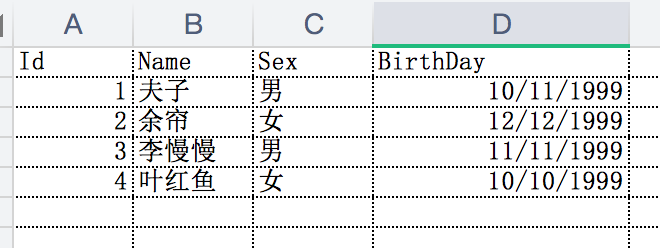# .Net Core Excel导入导出神器Npoi.Mapper

#### 前言

我们在日常开发中对Excel的操作可能会比较频繁，好多功能都会涉及到Excel的操作。在.Net Core中大家可能使用Npoi比较多，这款软件功能也十分强大，而且接近原始编程。但是直接使用Npoi大部分时候我们可能都会自己封装一下，毕竟根据二八原则，我们百分之八十的场景可能都是进行简单的导入导出操作，这里就引出我们的主角Npoi.Mapper了。

#### 简介

关于Npoi.Mapper看名字我们就知道，它并不是一款创新型的软件，而是针对Npoi的二次封装增强了关于Mapper相关的操作。秉承着使用非常简单的原则，不过这样能够满足我们日常开发工作中很大一部分应用场景。它的GitHub地址为https://github.com/donnytian/Npoi.Mapper，目前Star并不多才240多，但是确实是非常好用，这里强烈推荐一波。接下来我们就大概演示一下的它的使用。

#### 常规操作

Npoi.Mapper的主题内容包括两大块，一个是针对导入，一个是针对导出。接下来我们先来简单演示一下最基础的导入导出。首先我们新建一个Student类作为数据承载的载体，简单定义大致如下

public class Student
{
public int Id { get; set; }
public string Name { get; set; }
public string Sex { get; set; }
public DateTime BirthDay { get; set; }
}


<PackageReference Include="Npoi.Mapper" Version="3.5.1" />

##### 导出操作

static void Main(string[] args)
{
List<Student> students = new List<Student>
{
new Student{ Id = 1,Name="夫子",Sex="男",BirthDay=new DateTime(1999,10,11) },
new Student{ Id = 2,Name="余帘",Sex="女",BirthDay=new DateTime(1999,12,12) },
new Student{ Id = 3,Name="李慢慢",Sex="男",BirthDay=new DateTime(1999,11,11) },
new Student{ Id = 4,Name="叶红鱼",Sex="女",BirthDay=new DateTime(1999,10,10) }
};
//声明mapper操作对象
var mapper = new Mapper();
//第一个参数为导出Excel名称
//第二个参数为Excel数据来源
//第三个参数为导出的Sheet名称
//overwrite参数如果是要覆盖已存在的Excel或者新建Excel则为true，如果在原有Excel上追加数据则为false
//xlsx参数是用于区分导出的数据格式为xlsx还是xls
mapper.Save("Students.xlsx", students, "sheet1", overwrite: true, xlsx:true);
Console.WriteLine("执行完成");
}但是这样导出的Excel头信息为属性的名称，而且我们Student类中包含了一个时间字段BirthDay为DateTime类型，这个表示格式好像也不太符合我们常规的阅读习惯，那该怎么办呢？Npoi.Mapper为我们提供了两种处理方式，一种是通过Fluent的方式指定映射关系如下所示

var mapper = new Mapper();
//第一个参数表示导出的列名，第二个表示对应的属性字段
mapper.Map<Student>("姓名", s => s.Name)
.Map<Student>("学号", s => s.Id)
.Map<Student>("性别", s => s.Sex)
.Map<Student>("生日", s => s.BirthDay)
//格式化操作，第一个参数表示格式，第二表示对应字段
//Format不仅仅只支持时间操作，还可以是数字或金额等
.Format<Student>("yyyy-MM-dd", s => s.BirthDay);
mapper.Save("Students.xlsx", students, "sheet1", overwrite: true, xlsx:true);


public class Student
{
[Column("学号")]
public int Id { get; set; }
[Column("姓名")]
public string Name { get; set; }
[Column("性别")]
public string Sex { get; set; }
[Column("生日",CustomFormat = "yyyy-MM-dd")]
public DateTime BirthDay { get; set; }
}


static void Main(string[] args)
{
//构建Student集合
List<Student> students = new List<Student>
{
new Student{ Id = 1,Name="夫子",Sex="男",BirthDay=new DateTime(1999,10,11) },
new Student{ Id = 2,Name="余帘",Sex="女",BirthDay=new DateTime(1999,12,12) }
};
//构建Person集合
List<Person> persons = new List<Person>
{
new Person{ Id = 1,Name="陈某", Tel= 18833445566},
new Person{ Id = 2,Name="柯浩然", Tel = 15588997766}
};
var mapper = new Mapper();
//放入Mapper中
//第一个参数是数据集合，第二个参数是Sheet名称，第三个参数表示是追加数据还是覆盖数据
mapper.Put<Student>(students, "student",true);
mapper.Put<Person>(persons, "person",true);
mapper.Save("Human.xlsx");
}


[HttpGet]
{
List<Student> students = new List<Student>
{
new Student{ Id = 1,Name="夫子",Sex="男",BirthDay=new DateTime(1999,10,11) },
new Student{ Id = 2,Name="余帘",Sex="女",BirthDay=new DateTime(1999,12,12) },
new Student{ Id = 3,Name="李慢慢",Sex="男",BirthDay=new DateTime(1999,11,11) },
new Student{ Id = 4,Name="叶红鱼",Sex="女",BirthDay=new DateTime(1999,10,10) }
};

var mapper = new Mapper();
MemoryStream stream = new MemoryStream();
//将students集合生成的Excel直接放置到Stream中
mapper.Save(stream, students, "sheet1", overwrite: true, xlsx: true);
}


Save提供了几个重载方法，其中有一个就是将数据保存到Stream中，但是这里也踩到了一个坑，不过这个是Npoi的坑并不是Npoi.Mapper的坑，那就是Workbook.Write(stream)的时候会将stream关闭，如果继续操作这个Stream会报流已关闭的错误，而Npoi.Mapper的Save到Stream的方法恰恰是对这个方法的封装，这也是为何上面我没直接在File中直接返回Stream，而是将其转换为byte数组再返回的原因。

##### 导入操作

//Excel文件的路径
var mapper = new Mapper("Students.xlsx");
//读取的sheet信息
var studentRows = mapper.Take<Student>("sheet1");
foreach (var row in studentRows)
{
//映射的数据保留在value中
Student student = row.Value;
Console.WriteLine($"姓名:[{student.Name}],学号:[{student.Id}],性别:[{student.Sex}],生日:[{student.BirthDay:yyyy-MM-dd}]"); }  通过Take方法直接读取出来的是RowInfo集合，RowInfo是用来包装读取数据的包装类。通过它可以获取读取的行号，或读取过程中可能会出现异常情况，比如某一列读取失败，它会将列信息和报错信息记录下来，如果你不需要这些信息或者觉得遍历的时候比较麻烦想直接拿到需要的集合，可以通过如下方式转换一下 var studentRows = mapper.Take<Student>("sheet1"); //通过lambda获取到Student集合 var students = studentRows.Select(i => i.Value);  有的时候你可能不想定义一个POCO去接收返回的结果，而是想直接拿到读取信息，转换成你需要的数据格式。比如你想读取Excel中的数据，将结果转换为实体类直接入库，但是你不想定义一个专门的映射类去接收读取结果，这时候你需要一个动态类型去接收，而Npoi.Mapper恰恰提供了这样的功能，可以将Excel中的数据直接读取到dynamic中去，具体操作和上面类似 var mapper = new Mapper("Students.xlsx"); var studentRows = mapper.Take<dynamic>("sheet1"); foreach (var row in studentRows) { var student = row.Value; Console.WriteLine($"姓名:[{student.姓名}],学号:[{student.学号}],性别:[{student.性别}],生日:[{student.生日:yyyy-MM-dd}]");
}


[HttpPost]
{
//通过上传文件流初始化Mapper
//读取sheet1的数据
return mapper.Take<Student>("sheet1").Select(i=>i.Value);
}


#### 其他功能

##### 忽略操作

[Ignore]
public string IgnoredProperty { get; set; }


##### 合并单元格

[UseLastNonBlankValue]
public string ClassName { get; set; }

##### 自定义Map规则

/// <param name="columnName">对应Excel列的名称</param>
/// <param name="propertyName">对应实体的属性名称</param>
/// <param name="tryTake">该函数用于处理从Excel读取时针对单元格数据的处理</param>
/// <param name="tryPut">该函数用于处理将数据导出到Excel是针对源数据的处理</param>
public static Mapper Map<T>(this Mapper mapper, string columnName, string propertyName,
Func<IColumnInfo, object, bool> tryTake = null,
Func<IColumnInfo, object, bool> tryPut = null)
{
}


var mapper = new Mapper("Students.xlsx");
mapper.Map<Student>("性别", "Sex", (c, t) => {
Student student = t as Student;
student.Sex = c.CurrentValue == "男" ? "MAN" : "WOMAN";
return true;
}, null);


#### 总结

👇欢迎扫码关注我的公众号👇posted @ 2020-12-13 23:31  yi念之间  阅读(4929)  评论(53编辑  收藏